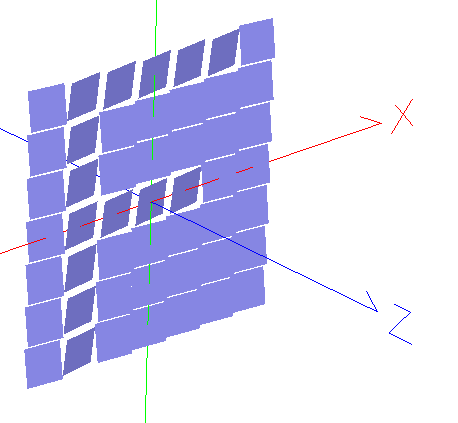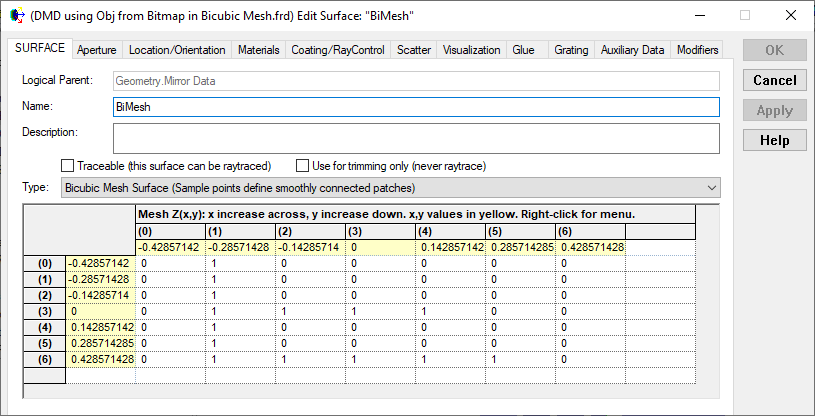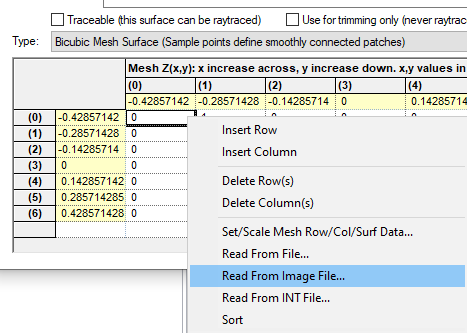# Digital Mirror Device (DMD)

## Introduction

Digital Mirror Devices (DMD)'s are used in a variety of display technologies and, at the simplest point of modelling, can be represented as an array of tilted mirrors.This article shows how to create such an array of mirrors using

• A bitmap to represent the on/off state for each mirror, in conjunction with a bicubic mesh surface to hold this data
• A script to write the DMD as an OBJ file (An OBJ is preferred as it imports as a single surface and is fast to ray trace).

## Example

The example is of a 7x7 array but can be scaled up to a larger number of pixels, including HD (1920x1080) resolutions, all that is required is a larger BMP file. The example file can be downloaded here:

DMD Example

To understand the steps that were taken to create this example we will discuss it in three parts.

Within the untraceable Custom Element node called "Mirror Data" is a BiCubic Mesh surface called "BiMesh". Open the surface to see the data used for the mirrors:This data was populated using the r-click menu within the grid to "Read from Image File..."Where the image used was a simple black and white BMP of a letter F (this bitmap is included in the example file).

Note that the settings of the "Read From Image File..." dialog box is what determines the X and Y size of the grid. In this case the 7x7 pixel bitmap and the default 0.5mm total semi width is what drives the center pixel values given in yellow around the mesh grid. The simple black and white BMP file used creates an array of 0's (Black) and 1's (White) which are used to represent the on/off state for each pixel/mirror.

### Part 2 - Running the Script

The embedded script called "Create OBJ DMD" was then ran to create the OBJ file. This script creates the OBJ object in the same location as the FRED document. The script:

• Sets the mirror tilt angle and fill factor
• Reads the BiMesh array to find the number of data points in X and Y (each will become a mirror or pixel) and calculates the pixel pitch and related values
• Defines an output file for the OBJ file
• Loops over each data point in the array of points and writes out four vertices which are then joined into two triangles to create the mirror. The mesh values are interpreted as on and off states, and the mirrors are given opposite tilts based on these values.